## Skipgram isn't Matrix Factorisation

The paper Neural Word Embeddings as Implicit Matrix Factorization of Levy and Goldberg was published in the proceedings of NIPS 2014 (pdf).  It claims to demonstrate that Mikolov’s Skipgram model with negative sampling is implicitly factorising the matrix of pointwise mutual information (PMI) of the word/context pairs, shifted by a global constant.  Although the paper is interesting and worth reading, it greatly overstates what is actually established, which can be summarised as follows:

Suppose that the dimension of the Skipgram word embedding is at least as large as the vocabulary.  Then if the matrices of parametersminimise the Skipgram objective, and the rows ofor the columns ofare linearly independent, then the matrix productis the PMI matrix shifted by a global constant.

This is a really nice result, but it certainly doesn’t show that Skipgram is performing (even implicitly) matrix factorisation.  Rather it shows that the two learning tasks have the same global optimum  – and even this is only shown when the dimension is larger than the vocabulary, which is precisely the case where Skipgram is uninteresting.

### The linear independence assumption

The authors (perhaps unknowingly) implicitly assume that the word vectors on one of the two layers of the Skipgram model are linearly independent.  This is a stronger assumption than what the authors explicitly assume, which is that the dimension of the hidden layer is at least as large as the vocabulary.  It is also not a very natural assumption, since Skipgram is interesting to us precisely because it captures word analogies in word vector arithmetic, which are linear dependencies between the word vectors!  This is not a deal breaker, however, since these linear dependencies are only ever approximate.

In order to see where the assumption arises, first recall some notation of the paper:The authors consider the case where the negative samples for Skipgram are drawn from the uniform distributionover the contexts, and write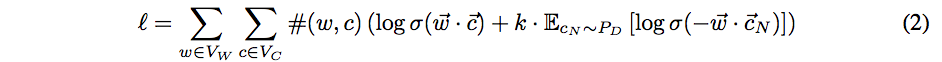for the log likelihood.  The log likelihood is then rewritten as another double summation, in which each summand (as a function of the model parameters) depends only upon the dot product of one word vector with one context vector: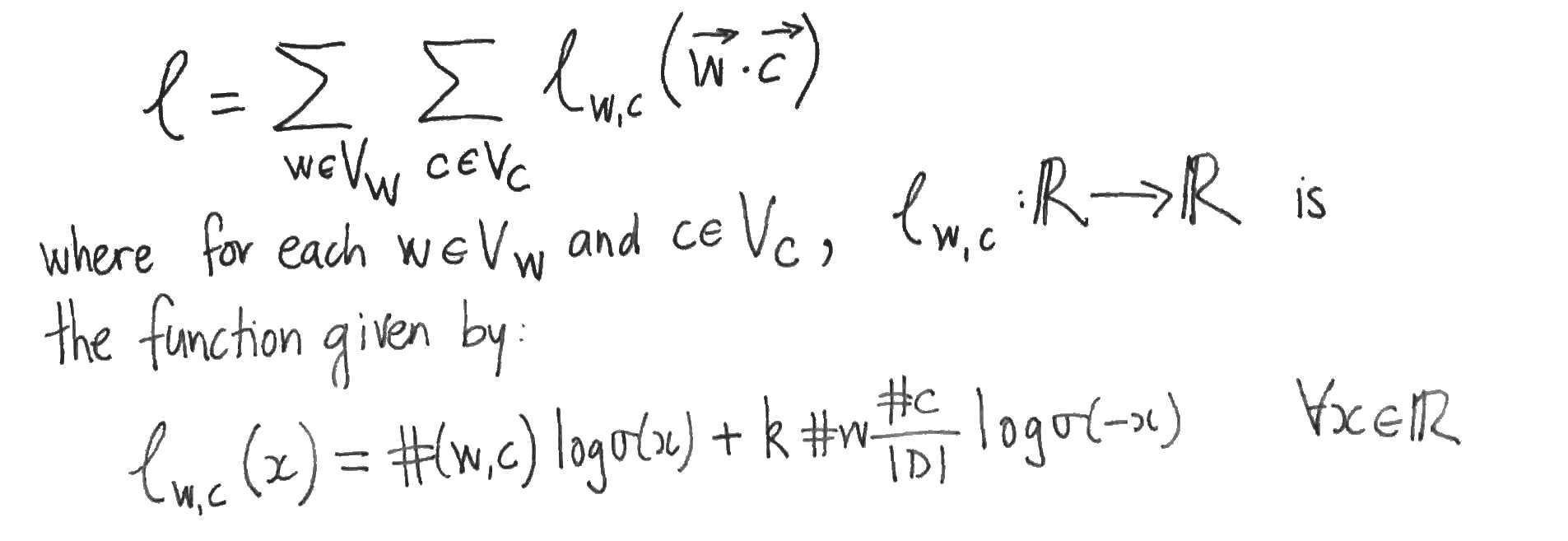The authors then suppose that the values of the parametersare such that Skipgram is at equilibrium, i.e. that the partial derivatives ofwith respect to each word- and content-vector component vanish.  They then assume that this implies that the partial derivatives ofwith respect to the dot products vanish also.  To see that this doesn’t necessarily follow, apply the chain rule to the partial derivatives:This yields systems of linear equations relating the partial derivatives with respect to the word- and content- vector components (which are zero by supposition) to the partial derivatives with respect to the dot products, which we want to show are zero.  But this only follows if one of the two systems of linear equations has a unique solution, which is precisely when its matrix of coefficients (which are just word- or context- vector components) has linearly independent rows or columns.  So either the family of word vectors or the family of context vectors must be linearly independent in order for the authors to proceed to their conclusion.

Word vectors that are of dimension the size of the vocabulary and linearly independent sound to me more akin to a one-hot or bag of words representations than to Skipgram word vectors.

### Skipgram isn’t Matrix Factorisation (yet)

If Skipgram is matrix factorisation, then it isn’t shown in this paper.  What has been shown is that the optima of the two methods coincide when the dimension is larger that the size of the vocabulary. Unfortunately, this tells us nothing about the lower dimensional case where Skipgram is actually interesting.  In the lower dimensional case, the argument of the authors can’t be applied, since it is then impossible for the word- or context- vectors to be linearly independent.  It is only in the lower dimensional case that the Skipgram and Matrix Factorisation are forced to compress the word co-occurrence information and thereby learn anything at all.  This compression is necessarily lossy (since there are insufficient parameters) and there is nothing in the paper to suggest that the two methods will retain the same information (which is what it means to say that the two methods are the same).

### Appendix: Comparing the objectives

To compare Skipgram with negative sampling to MF, we might compare the two objective functions.  Skipgram maximises the log likelihood(above). MF, on the other hand, typically minimises the squared error between the matrix and its reconstruction: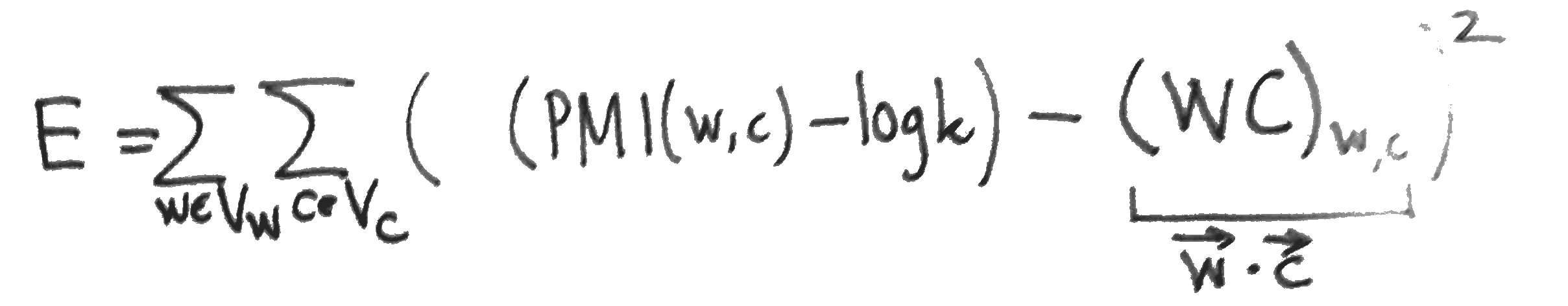The partial derivatives of, needed for a gradient update, are easy to compute: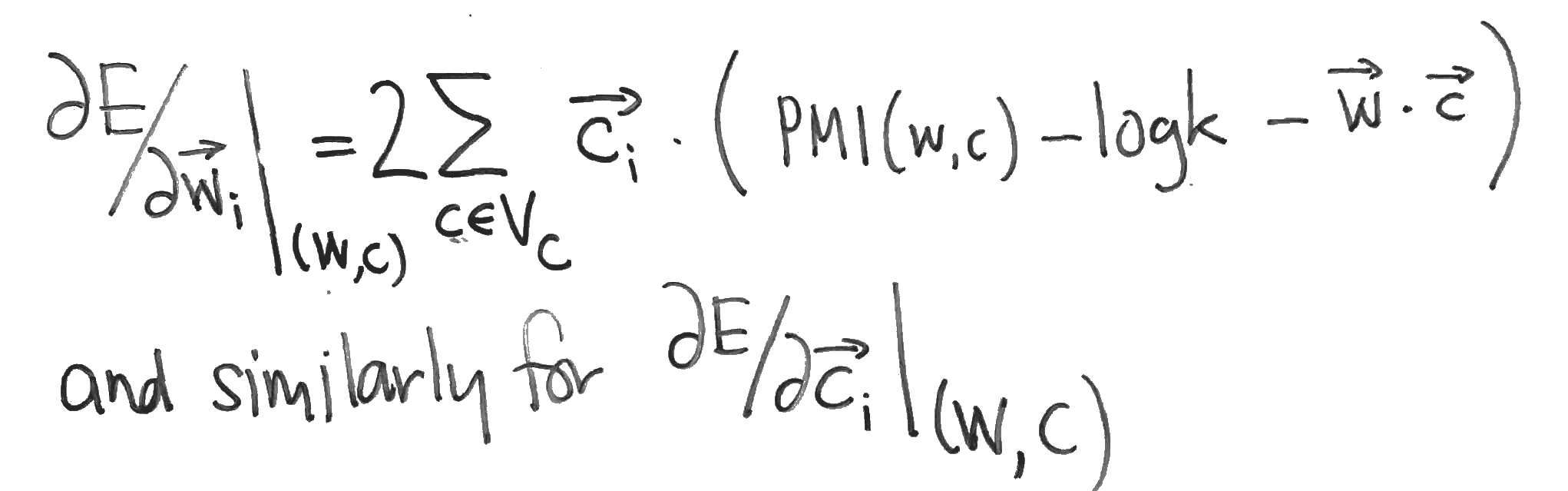Compare these with the partial derivatives of the Skipgram log-likehood, which can be computed as follows: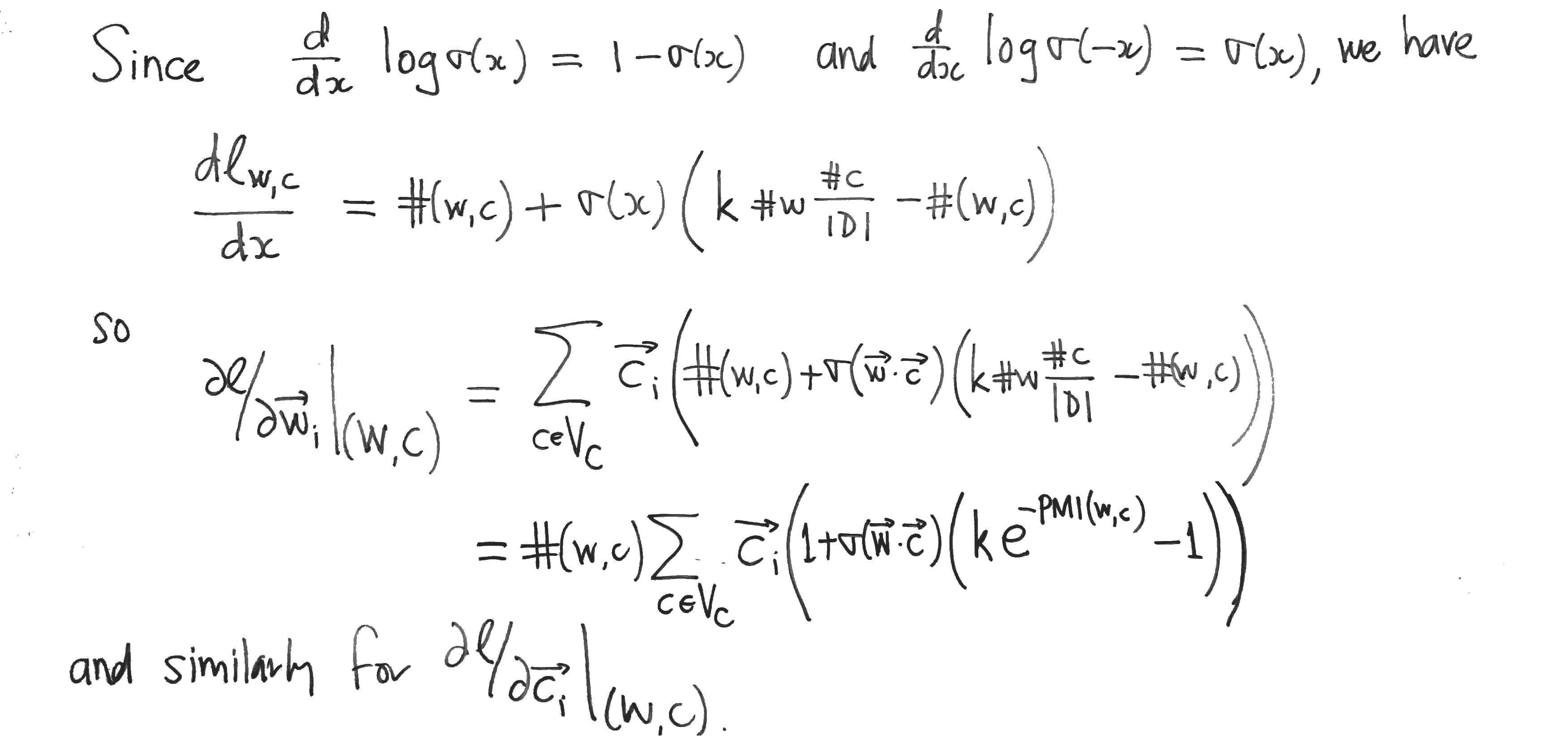## Does vector direction encode word frequency?

In a paper with Adriaan Schakel, we presented controlled experiments for word embeddings using pseudo-words. Performing these experiments in the case of word2vec CBOW showed that, in particular, the vector direction of any particular word changed only moderately when the frequency of the word was varied. Shortly before we released the paper, Schnabel et al presented an interesting paper at EMNLP, where (amongst other things), they showed that it was possible to distinguish rare from frequent words using logistic regression on the normalised word vectors, i.e. they showed that vector direction does approximately encode coarse (i.e. binary, rare vs. frequent) frequency information.  Here, I wanted to quickly report that the result of Schnabel et al. holds for the vectors obtained from our experiments, as they should. Below, I’ll walk through exactly what I checked.

I took the word vectors that we trained during our experiments. You can check our paper for a detailed account. In brief, we trained a word2vec CBOW model on popular Wikipedia pages with a hidden layer of size 100, negative sampling with 5 negative samples, a window size of 10, a minimum frequency of 128, and 10 passes through the corpus. Sub-sampling was not used so that the influence of word frequency could be more clearly discerned. There were 81k unigrams in the vocabulary. Then:

1. the word vectors were normalised so that their (Euclidean-) length was 1.
2. the frequency threshold of 5000 was chosen (somewhat arbitrarily) to define the boundary between rare and frequent words. This gave 8428 “frequent” words. A random sample of the same size of the remaining “rare” words was then chosen, so that the two classes, “rare” and “frequent”, were balanced. This yielded approximately 17k data points, where a data point is a normalised word vector labelled with either “frequent” (1) or “rare” (0).
3. the data points were split into training- and test- sets, with 70% of the data points in the training set.
4. a logistic regression model was fit on the training set. An intercept was fit, but this boosted the performance only slightly. No regularisation was used since the number of training examples wass high compared to the number of parameters.
5. The performance on the test set was assessed by calculating the ROC curve on the training and test sets and the accuracy on the test set.

Model performance
Consider the ROC curve below. We see from that fact that the test curve approximately tracks the training curve that the model generalises reasonably to unseen data. We see also from the closeness of the curves to the axes at the beginning and the end that the model is very accurate in detecting frequent words when it gives a high probability (bottom left of the curve) and at detecting infrequent words when it gives a low probability (top right).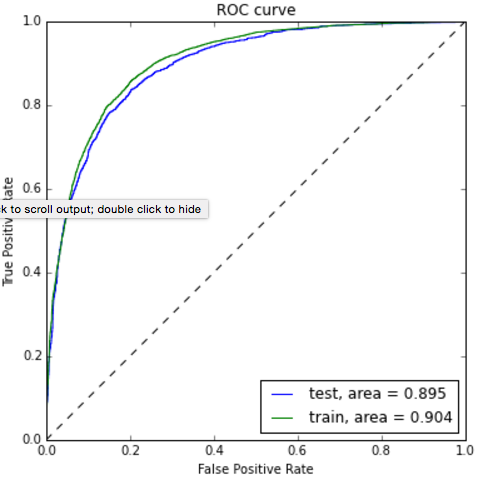The accuracy of the model on the test set was 82%, which agrees very nicely with what was reported in Schnabel et al., summarised in the following image: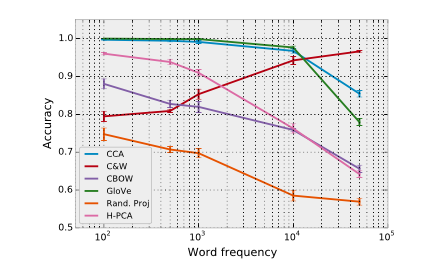The training corpus and parameters of Schnabel, though not reported in full detail (they had a lot of other things to report), seem similar. We know that their CBOW model was 50 dimensional, had a vocabulary of 103k words, and was trained on the 2008 Wikipedia.

## Musings on "adjectives as matrices"

The advantage of considering (e.g.) adjectives as transformations rather than points in space is that these transformations can be applied in unseen combinations. This counters one of Chomsky’s objections to statistical modelling of language, that is, that language is effectively infinite, whereas language models are trained on only a finite amount of data (so are humans, but humans are supposed to be born with a universal grammar). The case, considered by Baroni et al., of adjective as linear transform has a couple of disadvantages, however. The first that there are a large number of parameters to be learnt for each adjective, the second being that it doesn’t capture the near commutativity of adjectives, i.e. in most cases adjectives can be applied to a noun in different orders without significantly changing the meaning.

I can think of several approaches for enforcing the commutativity of adjective matrices:

1. simply using diagonal matrices (this reduces to one of the approaches already considered), or
2. penalising the off-diagonal elements via regularisation, or
3. interleaving existing parameter updates with updates that penalise (co-occurring?) adjective matrices for not commuting with one another, e.g. using the gradient of the matrix commutator## (Linear) Maps of the Impossible: Capturing semantic anomalies in distributional space

Eva Maria Vecchi, Marco Baroni and Roberto Zamparelli.

Presented at the workshop “Distributional Semantics and Compositionality” (2011) PDF

The authors attempt to use distributional models to distinguish between acceptable and “semantically deviant” adjective-noun combinations (an example of this distinction is given by “blue rose” vs “residential steak”). They hypothesise in particular that the length of the vector representation of the adjective-noun combination is an indication of its acceptability. Their reasoning for this hypothesis assumes that directions and in particular axes are interpretable in distributional models (this does not apply in the case of word2vec, at least). They further hypothesise that the combination will be spatially isolated with respect to the cosine similarity.

The distributional representation is derived from a POS-tagged and lemmatised corpus by considering sentence-internal co-occurrence between the vocabulary as a whole and the 10k most frequent nouns, verbs and adjectives, transformed via the “local mutual information” measure and reduced to rank 300 using PCA.

Different methods of transforming the noun representation using the adjective to obtain the adjective-noun combination are studied and the results are evaluated against human judgements of semantic deviance.